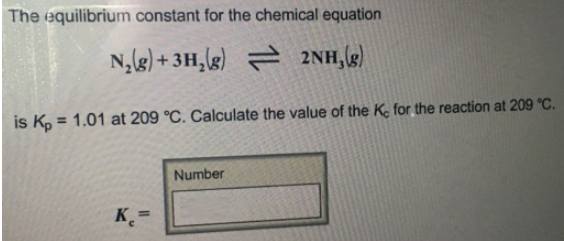# Problem: The equilibrium constant for the chemical equation N2 (g) + 3H2 (g) ⇌ 2NH3 (g) is Kp = 1 01 at 209°C. Calculate the value of the Kc for the reaction at 209°C.

###### FREE Expert Solution
97% (377 ratings)###### Problem Details

The equilibrium constant for the chemical equation

N2 (g) + 3H2 (g) ⇌ 2NH3 (g)

is Kp = 1 01 at 209°C. Calculate the value of the Kc for the reaction at 209°C.